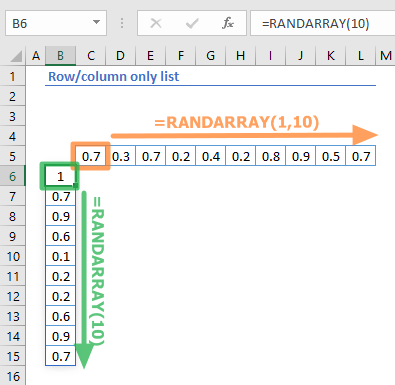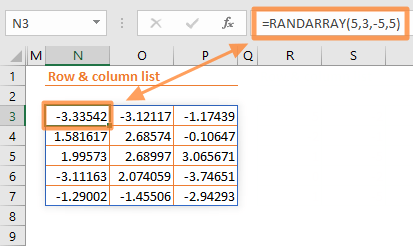The RANDARRAY function is a Math & Trigonometry formula that creates an array of randomly generated numbers. In this guide, we’re going to show you how to use the RANDARRAY function and also go over some tips and error handling methods.

The Dynamic Array functions can populate an array in a range of cells based on a formula. This behavior is called spilling and can help overcome the limitations of array formulas.

# Supported versions

• At the time of writing this article, Microsoft announced that this formula is currently only available to a number of select insider users. When it’s ready, the feature is planned for release for Office 365 users.

# Syntax

=RANDARRAY([rows],[columns],[min],[max],[whole_number])

# Arguments

 [rows] Optional. The number of rows of randomly generated numbers to be returned. The default value is 1. [columns] Optional. The number of columns of randomly numbers to be returned. The default value is 1. [min] Optional. The minimum number to be returned. The default value is 0. [max] Optional. The maximum number to be returned. The default value is 1. [whole_number] Optional. A Boolean value that specifies the type of numbers to return. ·         FALSE: Decimal number to 15 decimal places. (default) ·         TRUE: Whole number

Examples

## Row/column only list

=RANDARRAY(10)

=RANDARRAY(1,10)

formulas return one-dimensional arrays. Entering only a [rows] argument populates an array that has a certain number of rows in a single column. To populate an array across columns, enter a value for the [columns] argument as well.## Row & column array

=RANDARRAY(5,3,-5,5)
formula returns a two-dimensional array within the specified limits. While the values of the [rows] and columns] arguments (5 and 3 here) determine the size of return array, the [min] and [max] values specify the top and bottom limits for the random number generation.## Random Whole Numbers

=RANDARRAY(5,3,-5,5,TRUE)
formula users the [whole_number] parameter. Like in the previous example, a two-dimensional array of random numbers will be generated in specified limits. However, in this scenario the generated numbers numbers are whole numbers (integers).# Tips

• The RANDARRAY function is a volatile function. This means that it re-calculates the results with every calculation and this can affect the performance of the workbook.
• If you enter nothing for the parameters, the RANDARRAY function acts like the RAND function.
=RANDARRAY()
• You can use RANDARRAY as a substitute for the RANDBETWEEN. This requires omitting the [rows] and [columns] arguments and setting [whole_number] to TRUE. For example, to return values between 5 and 10, use
=RANDARRAY(,,5,10,TRUE)
• You can combine the RANDARRAY with the SORTBY function to shuffle an array of values.

# Issues

## #SPILL!

If there isn't enough space for adding the results below the formula, you will see a #SPILL! error. Excel marks the target range with dashed lines. Clear the contents of the cells in this range, and Excel will automatically update the results.## #VALUE!

• If the [min] is greater than or equal to the [max] you will get a #VALUE! error.
• If any of the argument is not a numeric value, you will get a #VALUE! error.